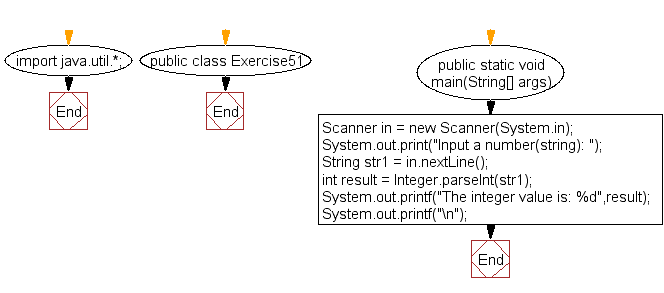﻿ Java exercises: Convert a string to an integer in Java - w3resource# Java Exercises: Convert a string to an integer in Java

## Java Basic: Exercise-51 with Solution

Write a Java program to convert a string to an integer in Java.

Sample Solution:

Java Code:

``````import java.util.*;
public class Exercise51 {

public static void main(String[] args)
{
Scanner in = new Scanner(System.in);
System.out.print("Input a number(string): ");
String str1 = in.nextLine();
int result = Integer.parseInt(str1);
System.out.printf("The integer value is: %d",result);
System.out.printf("\n");
}
}
```
```

Sample Output:

```Input a number(string): 25
The integer value is: 25
```

Flowchart:Java Code Editor:

What is the difficulty level of this exercise?

Test your Programming skills with w3resource's quiz.

﻿

## Java: Tips of the Day

countOccurrences

Counts the occurrences of a value in an array.

Use Arrays.stream().filter().count() to count total number of values that equals the specified value.

```public static long countOccurrences(int[] numbers, int value) {
return Arrays.stream(numbers)
.filter(number -> number == value)
.count();
}
```

Ref: https://bit.ly/3kCAgLb